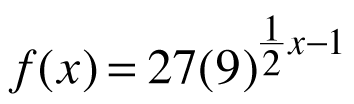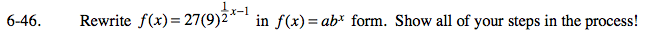### Home > PC > Chapter 6 > Lesson 6.1.3 > Problem6-46

6-46.

Rewritein f(x) = abx form. Show all of your steps in the process! Homework Help ✎Change the 27 and the 9 to numbers with base 3.

$f(x)=3^{3}(3^2)^{\left(\frac{1}{2}x-1\right)}$

Use the power to a power property of exponents.

f(x) = 33(3)x − 2

Use the multiplication property of exponents.

f(x) = (3)3 + x − 2

Simplify.

f(x) = (3)x + 1

Put in the abx form.

f(x) = 3(3)x